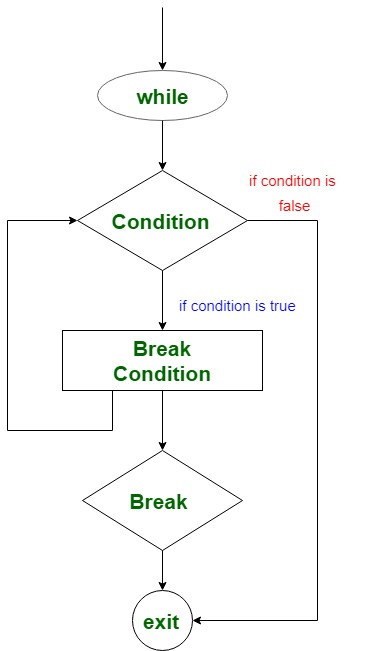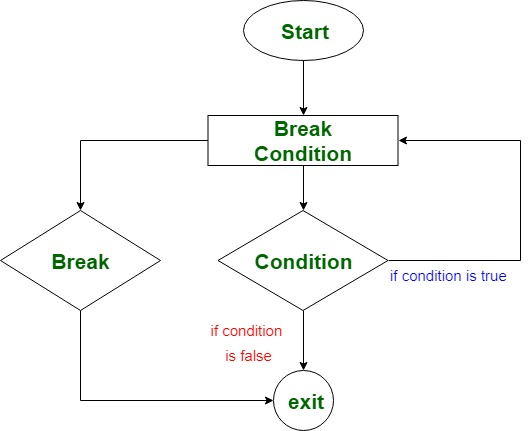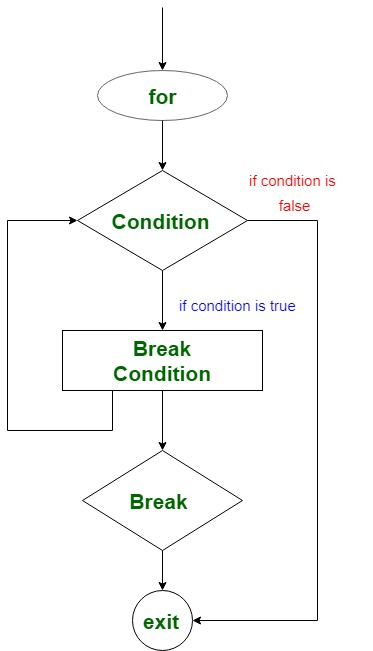# Kotlin Unlabelled break

• Last Updated : 09 Aug, 2021

When we are working with loops and want to stop the execution of loop immediately if a certain condition is satisfied, in this case, we can use either break or return expression to exit from the loop.
In this article, we will discuss learn how to use break expression to exit a loop. When break expression encounters in a program it terminates to nearest enclosing loop.
There are two types of break expression in Kotlin:
We are going to learn how to use unlabelled break expression in while, do-while and for loop.

### Use of unlabelled break in while loop

Unlabelled break is to used to exit the loop when it satisfies a specific condition without checking the test expression. Then, transfers the control to the following statement of while block.
Syntax of break in while loop –

```while(test expression) {
// code to run
if(break condition) {
break
}
// another code to run
}```

Flowchart-Kotlin program to find the sum of integers from 1 to 10.

## Java

 `fun main(args: Array) {``    ``var sum = ``0``    ``var i = ``1``    ``while``(i <= Int.MAX_VALUE) {``        ``sum += i``        ``i++``        ``if``(i == ``11``) {``            ``break``        ``}``    ``}``    ``print(``"The sum of integers from 1 to 10: \$sum"``)``}`

Output:

`The sum of integers from 1 to 10: 55`

In the above program, we calculate the sum of integers from 1 to 10 with the help of while loop and break expression. Take a variable sum and initialize it with 0. Another variable i to iterate through the loop and initialize it with 1.
Now, iterator continues from (i = 1) and execute sum statement. When the iterator value i becomes 11 then it executes break expression and exit the loop without checking the test expression (i <= Int.MAX_VALUE). Then, control passes to the following statement print() of while block and it prints the sum of integers = 55

### Use of unlabelled break in do-while loop –

In do-while loop also we can use the break expression to exit the loop without checking the test expression.
Syntax for break in do-while loop –

```do {
//code to run
if(break condition) {
break
}
while(test expression)```

Flowchart-Kotlin program to print the elements of an array

## Java

 `fun main(args: Array) {``    ``var names = arrayOf(``"Earth"``,``"Mars"``,``"Venus"``,``"Jupiter"``,``"Saturn"``,``"Uranus"``)``    ``var i = ``0`` ` `    ``do``{``        ``println(``"The name of \$i th planet: "``+names[i])``        ``if``(names[i]==``"Jupiter"``) {``            ``break``        ``}``        ``i++``    ``}``while``(i<=names.size)``}`

Output:

```The name of 0 th planet: Earth
The name of 1 th planet: Mars
The name of 2 th planet: Venus
The name of 3 th planet: Jupiter```

In the above program, we traverse the array to print names of planets. First of all, initialize an array names with planet names and i is the iterator for test expression. We calculate the size of an array using names.size
do block first print the element of the array and every time the value of the array at any index is compared with “Jupiter”. If it does match, then increment the iterator and execute again. If matches then execute the break expression and exit the do-while loop without checking for the test expression.

### Use of unlabelled break in for loop –

We can use break expression while traversing the for loop with in an array or string.
The syntax of break in for loop-

```for(iteration through iterator) {
// code to run
if(break condition){
break
}
}```

Flowchart-Kotlin program to print string upto a particular character
In the below program we traverse the string to break at a particular position by comparing the char value. First of all, initialize an array name with value “GeeksforGeeks”. Then a for loop to traverse using an iterator i. It prints the char value and compares at each position with char ‘s’. If matches, then exit the loop and transfer the control to the following statement.

## Java

 `fun main(args: Array) {`` ` `    ``var name = ``"GeeksforGeeks"``    ``for` `(i in name){``     ``print(``"\$i"``)``          ``if``(i == ``'s'``) {``            ``break``          ``}``    ``}``}`

Output:

`Geeks`

My Personal Notes arrow_drop_up